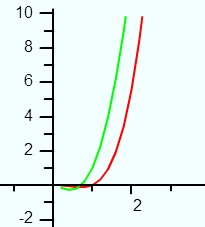# Single Variable Calculus: Definition

Single variable calculus deals with functions of one variable. For example,

f(x) = 2x

has one variable x, so it would be included in this type of calculus. While x is the most common variable, you’ll also come across t (for time) z (for complex numbers) and a variety of other single variables.

## Why is it called Single Variable Calculus?

It’s called “single variable calculus” to set it apart from other types of calculus. Calculus is a very broad umbrella term that has many subdivisions. These include:

Many of these subdivisions overlap with each other. For example, differential calculus involves working with derivatives. So, if you have a one-variable function like f(x) = x2, and you find a derivative, that falls into differential calculus and single variable calculus.

## What Subjects are in Single Variable Calculus?Graph of y=x3 ln x (red line). When you find the derivative of y = x3 ln x you get x2 + 3x2 ln x (red line)
One variable calculus is an introduction to calculus, where you’ll learn about derivatives and integrals.

A derivative is the slope of a tangent line at a point. This information tells you how fast, or how slow, a system is changing. This can be applied to many thousands of real life situations. For example, it could help you answer questions like:

• How fast is the chemical mixture changing at any point in time?
• If I shoot a rocket into the sky, at which point is it at its maximum acceleration?

The other arm of single variable calculus is integration. An integral is the space under the graph of a function; It’s the reverse of a derivative. Integrals help you to find things like displacement (with displacement functions), time passed, and velocity (using the velocity function).

You’ll also be introduced to a wide variety of functions, including Transcendental Functions and parametric functions. You’ll work with Curve Sketching, and (usually) polar coordinate systems. At the end of the course, you might be introduced to differential equations.

## References

Keisler, H. Elementary Calculus: An Approach Using Infinitesimals. Dover Publications; 3rd edition (February 15, 2012).

CITE THIS AS:
Stephanie Glen. "Single Variable Calculus: Definition" From StatisticsHowTo.com: Elementary Statistics for the rest of us! https://www.statisticshowto.com/single-variable-calculus/